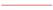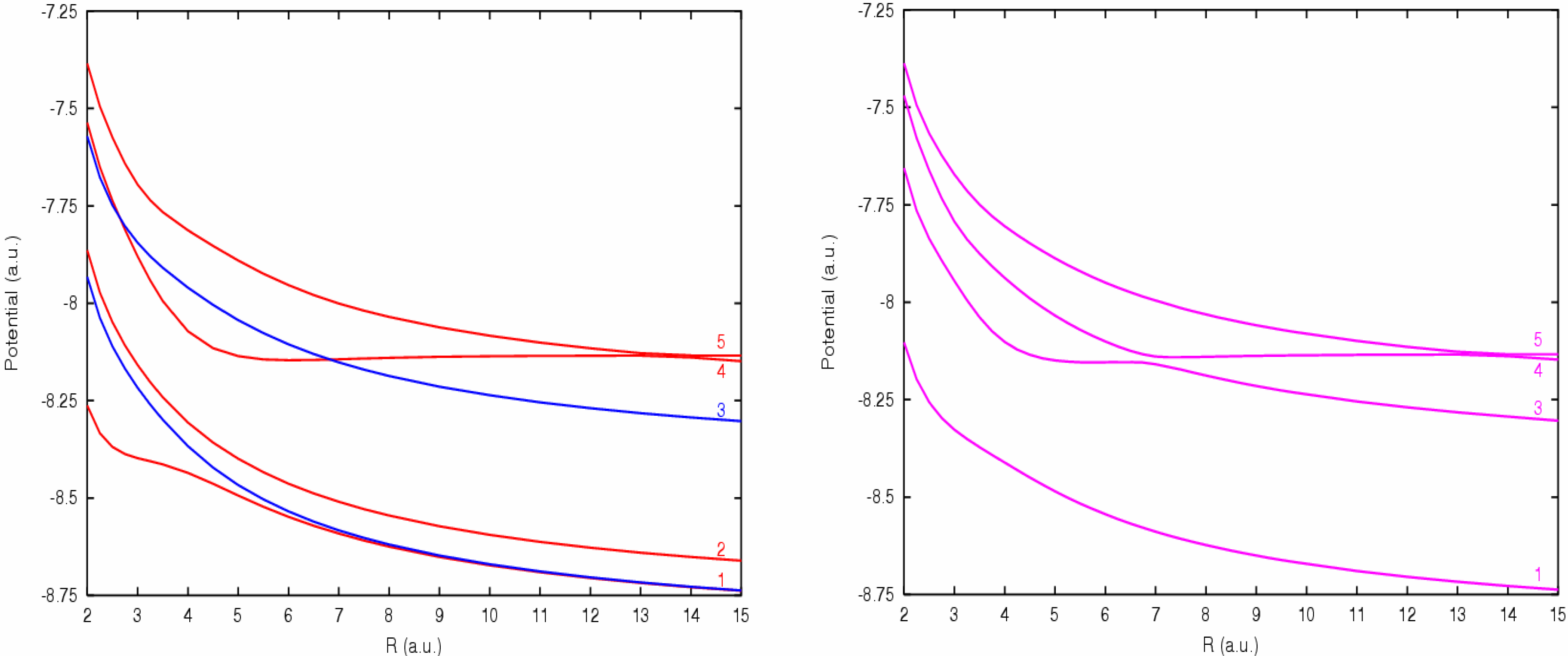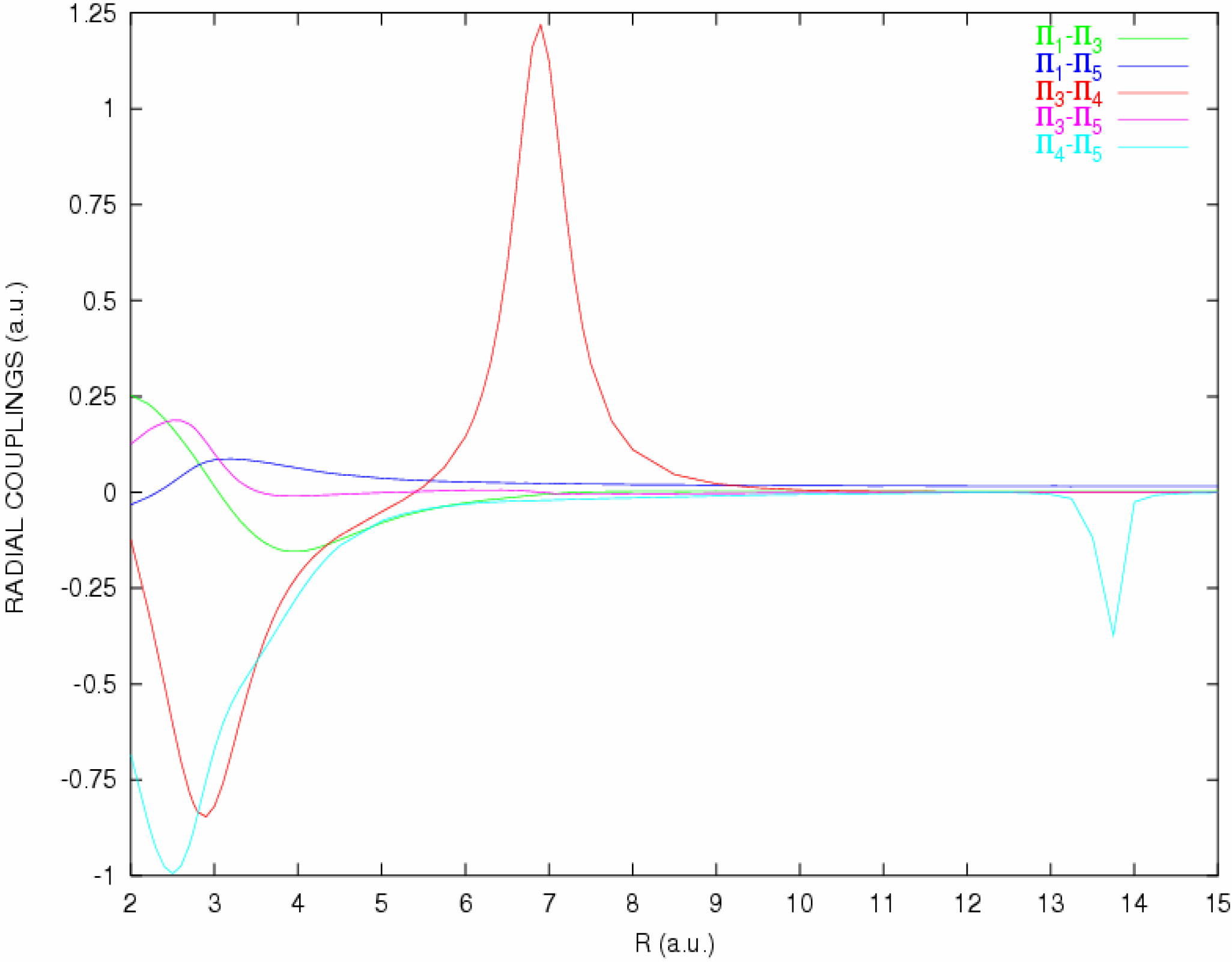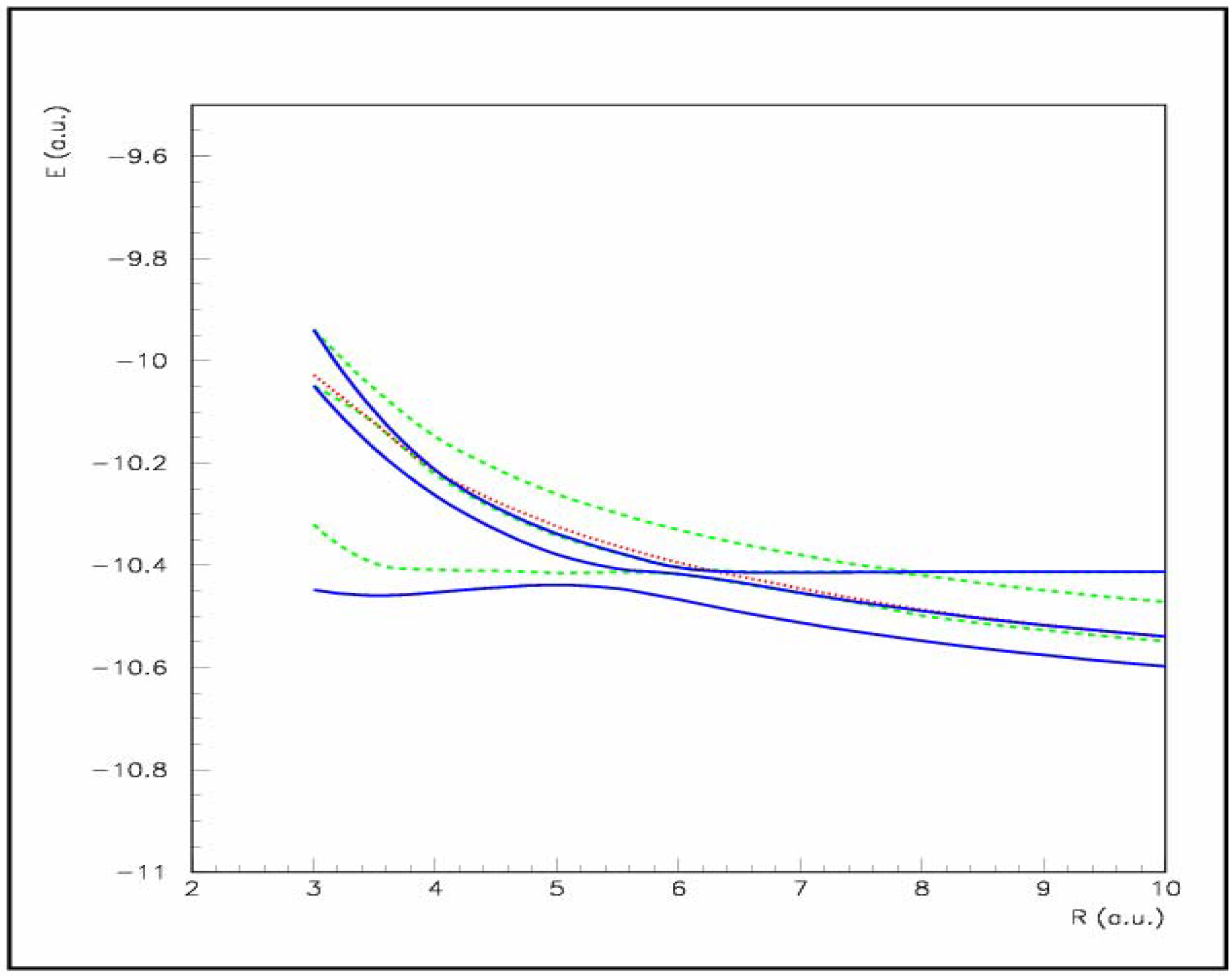Article

# State Selective Electron Capture in the Collision of S3+ Ions in Atomic Hydrogen and Helium

1
Laboratoire de Spectrométrie Ionique et Moléculaire, UMR 5579, CNRS et Université Lyon I, 43 Bd. du 11 Novembre 1918, 69622 Villeurbanne Cedex, France
2
Department of Theoretical Physics and Mathematical Methods, Gdańsk University of Technology, ul. Narutowicza 11/12, Pl-80-952 Gdańsk, Poland
*
Author to whom correspondence should be addressed.
Int. J. Mol. Sci. 2004, 5(11), 265-275; https://doi.org/10.3390/i5110265
Received: 8 September 2004 / Revised: 9 November 2004 / Accepted: 9 November 2004 / Published: 30 November 2004

## Abstract

A full theoretical treatment including an ab-initio molecular calculation of the potential energy curves and couplings followed by a semi-classical collision dynamics has been performed for the one-electron capture by S3+ ions in collision with atomic hydrogen. The present paper completes a previous letter  and displays the full results concerning this process in order to provide a detailed understanding of the mechanism. These calculations show evidence of the predominance of the S2+(3s23p3d)3F° capture level, already pointed out by translational energy spectroscopy experiments and confirms experimental measurements. A compared study of the behaviour of the S3+ projectile colliding both hydrogen and helium targets is also presented.

## Introduction

The charge transfer recombination involving low-energy multiply charged ions in collision with atomic or molecular targets is an important process in astrophysical plasmas for many low charged ions whose emission lines are used to provide direct information on the ionization structure of astrophysical objects. An accurate understanding of the emergent spectra requires a detailed modelling of the atomic and molecular processes affecting conditions in the gas, as collisional excitation, photodissociation, but also charge transfer processes which appear to be essential for the determination of the ion abundances and ionization balance of the plasmas [1,2,3].
Second row ions, such as silicon and sulfur play an important role in the reactions occurring in the interstellar medium [4,5], nevertheless, relatively few studies have been devoted to charge transfer processes involving sulfur ions [6,7,8,9,10,11,12]. Experimental measurements using the translational energy spectroscopy technique have been carried out by Wilson et al.  on state-selective electron capture by S3+ ions in H, H2 and He at impact energies within the range 2.4-9.0 keV. They show evidence of an S2+ triplet state, identified as the S2+(3s23p3d)3F° level, lying 15.03 eV above the S2+ ground state in agreement with atomic data , which appears to be the dominant capture state in the S3+ + H collision system over the entire range of impact energies investigated. The multichannel Landau-Zener approach fails to reproduce the experimental spectra, in particular it largely underestimates the capture on peak A assigned to the S2+(3F°) + H+ level. We present here a full detailed study of the S3+(3s23p) + H electron capture mechanism, including all the triplet and singlet states correlated to the 1,3Σ and 1,3Π entry channels and involved in the process by means of radial or rotational coupling matrix elements. The calculation has been performed for the ground state S3+(3s23p)2P° + H entry channel only ; the metastable ion S3+(3s3p2)4P evidenced in the ion beam, has not been taken into account in this study. These results may be compared to our previous work on the S3+ + He charge transfer process  using the CIPSI algorithm for the molecular treatment followed by a semi-classical collision dynamics.

## Molecular calculations of the S3+(3s23p)2P° + H collisional system

#### A. Potential energy curves

The potential energy curves have been carried out using the MOLPRO suite of ab initio programs  at the state average CASSCF-MRCI level of theory. The active space includes the n=3 and n=4(sp) orbitals for the sulfur element, and the 1s orbital for hydrogen. A pseudopotential has been used to take account of the core electrons of the sulfur atom. Tests have been performed for the choice of this pseudopotential and the basis of atomic orbitals. The ECP10sdf 10 core-electron relativistic pseudopotential has been used for the sulfur atom  with correlation-consistent aug-cc-pVQZ basis sets of Dunning for sulfur and hydrogen . Such a basis set shows a quite good agreement with experiment  for the atomic energy levels with a discrepancy in the range 0.01-0.14 eV (Table I).
Table I. Comparison of atomic energy levels with experiment  (in eV).
Table I. Comparison of atomic energy levels with experiment  (in eV).
MRCI calculationExperiment
S3+(3s23p)235.1134.98
S2+(3s3p3)3S° + H+ 17.05
S2+(3s3p3)3P° + H+12.3112.17
S2+(3s3p3)3D° + H+10.3410.35
S2+(3s23p2)3P + H+0.00.0
The spin-orbit effects may be neglected in the energy range of interest so triplet and singlet manifolds can be considered separately. From the atomic energy level data, it appears clearly that the S2+(3s23p3d)3P° electron capture level cannot account for the present charge transfer mechanism. Effectively, the asymptotic energy difference between the entry channel 1,3Π, 1,3Σ+ {S3+(3s23p)2P° + H(1s)2S} and the capture levels correlated to the {S2+(3s23p3d)3P° + H+1S} configuration is shown in tables to be equal to 3.65 eV, quite different from the energy defect of 6.2 eV observed experimentally for peak A. From the asymptotical repulsion term, this energy difference would lead to a long range interaction with an avoided crossing around R=15 a.u. which could hardly explain an important population of the corresponding capture channel. It seems thus likely, as proposed by Wilson et al. , to consider the capture level S2+(3s23p3d)3F°, accessible from the entry channel 1,3Π, 1,3Σ+ {S3+(3s23p)2P° + H(1s)2S}. The collisional system appears thus relatively complex, and a great number of states have to be taken into account in both the triplet and singlet manifold including one-electron capture processes as well as reactions of capture and excitation of the 3s electron:
 S3+(3s23p)2P° + H(1s)2S 3Π, 3Σ+ 1Π, 1Σ+ entry channel S2+(3s3p3)1P° + H+1S 1Π, 1Σ+ S2+(3s3p3)3S° + H+1S 3Σ- S2+(3s23p3d)3F° + H+1S 3Φ, 3Π, 3Δ, 3Σ+ S2+(3s3p3)1D° + H+1S 1Π, 1Δ, 1Σ- S2+(3s3p3)3P° + H+1S 3Π, 3Σ+ S2+(3s3p3)3D° + H+1S 3Π, 3Δ, 3Σ- S2+(3s23p2)1S + H+1S 1Σ+ S2+(3s23p2)1D + H+1S 1Π, 1Δ, 1Σ+ S2+(3s23p2)3P + H+1S 3Π, 3Σ-
The potentials correlated to the S3+(3s23p)2P° + H entry channel are displayed in Figures 1a,b for singlet states, and in Figure 2a,b for triplet ones. The Σ- levels have not been taken into account.
Figures 1a,b. Adiabatic potential energy curves of the 1Σ+, 1Δ and 1Π states of the S3+(3s23p) + H collisional system.1Σ+ states;1Δ states;1Π states. 1, 1Σ+, 1Δ and 1Π states corresponding to {S2+(3s23p2)1D + H+1S}; 2, 1Σ+ state corresponding to {S2+(3s23p2)1S + H+1S}; 3, 1Δ and 1Π states corresponding to {S2+(3s3p3)1D° + H+1S}; 4, 1Σ+ and 1Π states corresponding to {S2+(3s3p3)1P° + H+1S}; 5, 1Σ+ and 1Π states corresponding to {S3+(3s23p)2P° + H(1s)2S}, entry channel.
Figures 1a,b. Adiabatic potential energy curves of the 1Σ+, 1Δ and 1Π states of the S3+(3s23p) + H collisional system.1Σ+ states;1Δ states;1Π states. 1, 1Σ+, 1Δ and 1Π states corresponding to {S2+(3s23p2)1D + H+1S}; 2, 1Σ+ state corresponding to {S2+(3s23p2)1S + H+1S}; 3, 1Δ and 1Π states corresponding to {S2+(3s3p3)1D° + H+1S}; 4, 1Σ+ and 1Π states corresponding to {S2+(3s3p3)1P° + H+1S}; 5, 1Σ+ and 1Π states corresponding to {S3+(3s23p)2P° + H(1s)2S}, entry channel.For the singlet manifold, the potential energy curves show clearly a relatively long range crossing, around R=13 a.u., between both the 1Π and 1Σ+ entry channels and the corresponding capture levels of the {S2+(3s3p3)1P° + H+1S} configuration. This crossing is associated with an energy defect of about 4.0 eV and cannot be attributed to any experimental peak, in fact such a long range crossing might be considered as almost inactive in the charge transfer mechanism. However, an efficient avoided crossing may be considered near R=7 a.u. corresponding to the interaction with the 1Π {S2+(3s3p3)1D° + H+1S} level, it corresponds to an energy defect of 8.2 eV and may contribute to the experimental peak B.
According to the statistical weight, the triplet manifold accounts for ¾ of the population of the ground state entry channel, against ¼ for the singlet manifold, and appears thus to be preponderant in the charge transfer mechanism. The triplet potential energy curves show several avoided crossings which may play a quite important role. Two short range avoided crossings are observed with the 3Π, 3Σ+ levels corresponding to the {S2+(3s3p3)3P° + H+1S} configuration, around R=6 a.u.; they are associated with an energy defect of 9.19 eV and may contribute to the experimental peak B. Another interaction appears with the 3Π {S2+(3s3p3)3D° + H+1S} level, around R=5 a.u., which may contribute to peak C, with an energy defect of 11.16 eV. But the most important feature appears for the state quoted 4 in Fig. 2a,b which exhibits an avoided crossing around R=9.7 a.u., corresponding to an energy defect of 5.6 eV, with the entry channel in both 3Σ+ and 3Π symmetries. This channel is associated simultaneously with state symmetry 3Σ+, 3Δ, 3Π, and 3Φ and may thus be attributed without any ambiguity to the {S2+(3s23p3d)3F° + H+1S} configuration, confirming the experimental deduction of Wilson et al. . The energy defect appears however slightly lower than evidenced for the experimental peak A. From our ab-initio MRCI calculations, the S2+(3F°) capture level appears at an energy of 15.8 eV above the S2+(3s23p2)3P ground state, a somewhat higher value than the 15.03 eV experimental value of Wilson et al.  and the 15.8 eV value given by the NIST Database , although the set of molecular calculations appears to be globally in good accordance with the tabulated energies as shown in Table II.
Table II. Comparison with experiment  for asymptotic energy levels (in a.u.)
Table II. Comparison with experiment  for asymptotic energy levels (in a.u.)
MRCI calculationExperiment
S3+(3s23p)2P° + H(1s)2S0.00.0
S2+(3s3p3)3S° + H+ -0.1572
S2+(3s3p3)1P° + H+-0.1477-0.1628
S2+(3s3p3d)3F° + H+-0.2063-0.2313a
S2+(3s3p3)1D° + H+-0.3019-0.3117
S2+(3s3p3)3P° + H+-0.3379-0.3363
S2+(3s3p3)3D° + H+-0.4105-0.4032
S2+(3s23p2)1S + H+-0.6601-0.6625
S2+(3s23p2)1D + H+-0.7371-0.7347
S2+(3s23p2)3P + H+-0.7905-0.7837
a ref. 
Figures 2a,b. Adiabatic potential energy curves of the 3Σ+, 3Δ, 3Π and 3Φ states of the S3+(3s23p) + H collisional system.3Σ+ states;3Δ states;3Π states;Φ state. 1, 3Π state corresponding to {S2+(3s23p2)3P + H+1S}; 2, 3Δ and 3Π states corresponding to {S2+(3s3p3)3D° + H+1S}; 3, 3Σ+ and 3Π states corresponding to {S2+(3s3p3)3P° + H+1S}; 4, 3Σ+, 3Δ, 3Π and 3Φ states corresponding to {S2+(3s23p3d)3F° + H+1S}; 5, 3Σ+ and 3Π states corresponding to {S3+(3s23p)2P° + H(1s)2S}, entry channel.
Figures 2a,b. Adiabatic potential energy curves of the 3Σ+, 3Δ, 3Π and 3Φ states of the S3+(3s23p) + H collisional system.3Σ+ states;3Δ states;3Π states;Φ state. 1, 3Π state corresponding to {S2+(3s23p2)3P + H+1S}; 2, 3Δ and 3Π states corresponding to {S2+(3s3p3)3D° + H+1S}; 3, 3Σ+ and 3Π states corresponding to {S2+(3s3p3)3P° + H+1S}; 4, 3Σ+, 3Δ, 3Π and 3Φ states corresponding to {S2+(3s23p3d)3F° + H+1S}; 5, 3Σ+ and 3Π states corresponding to {S3+(3s23p)2P° + H(1s)2S}, entry channel.#### B. Radial and rotational coupling matrix elements

The radial coupling matrix elements between all pairs of states of the same symmetry have been calculated by means of the finite difference technique:
$g K L ( R ) = 〈 ψ K | ∂ / ∂ R | ψ L 〉 = lim Δ → 0 1 Δ 〈 ψ K ( R ) | ψ L ( R + Δ ) 〉 ,$
with the parameter Δ=0.0012 a.u. as previously tested. The sulfur nucleus has been chosen as origin of electronic coordinates. The main matrix elements are displayed in Figure 3 for the singlet manifold, and in Figures 4a,b for the triplet manifold.
Figure 3. Radial coupling matrix elements between 1Π states of the S3+(3s23p) + H collisional system (see labels in Figures 1a,b).
Figure 3. Radial coupling matrix elements between 1Π states of the S3+(3s23p) + H collisional system (see labels in Figures 1a,b).They present the same features as exhibited by the potential energy curves. For the singlet manifold, the couplings between the 1Σ+ and 1Δ states remain small for all distances, lower than 0.2 a.u., in relation with the smooth interactions observed between the corresponding potentials. The only sharp peak is observed between 1Π states, around R=7 a.u and about 1.25 a.u. high, in correspondence with the avoided crossing between the 1Π entry channel and the 1Π {S2+(3s3p3)1D° + H+1S} capture channel.
For the triplet manifold, 3Σ+ states show two peaks, one around R=6 a.u. corresponding to the avoided crossing between the entry channel and the 3Σ+{S2+(3s3p3)3P° + H+1S} capture level associated with the experimental peak B, and a sharper one, around R=9.7 a.u., in correspondence with the strong avoided crossing between the entry channel and the 3Σ+ {S2+(3s23p3d)3F° + H+1S} excited one-electron capture level. This radial coupling matrix element reaches 2.75 a.u. and is clearly the most important one for the charge transfer process, associated with the experimental peak A. Radial coupling between 3Δ states remain small for all internuclear distances. The 3Π levels show also such a sharp peak for the radial coupling corresponding to the avoided crossing with the 3Π {S2+(3s23p3d)3F° + H+1S} capture channel. It reaches even 3.5 a.u. and is markedly higher that the other radial coupling matrix elements. The coupling associated with the avoided crossing between the 3Π entry channel and the 3Π{S2+(3s3p3)3P° + H+1S} level presents a peak, 2.5 a.u. high, around R=6 a.u. and the interaction with the 3Π {S2+(3s3p3)3D° + H+1S} capture channel shows a smooth variation of the radial coupling matrix element with a hump around R=5 a.u..
Figures 4a,b. Radial coupling matrix elements between 3Σ+, 3Δ and 3Π states of the S3+(3s23p) + H collisional system (see labels in Figures 2a,b).
Figures 4a,b. Radial coupling matrix elements between 3Σ+, 3Δ and 3Π states of the S3+(3s23p) + H collisional system (see labels in Figures 2a,b).The rotational matrix elements $〈 ψ K | i L y | ψ L 〉$ between states of angular moment ΔΛ=±1 have been calculated directly from the quadrupole moment tensor. The coupling matrix elements for the triplet manifold are displayed in Figure 5. Rotational couplings between Σ-Π and Π-Δ are zero at long range for states corresponding to different configurations, as shown for example for the coupling between 3Σ+{S3+(3s3p3)3P° + H+1S} and 3Π{S2+(3s3p3)3D° + H+1S} levels. The couplings corresponding to the same configuration reach the asymptotical value 1.0 a.u., as shown for the 3Σ+-3Π couplings corresponding to the {S3+(3s23p)2P° + H(1s)2S} and the {S2+(3s3p3)3P + H+1S} configurations, as well as to the 3Π-3Δ rotational couplings associated with the {S2+(3s3p3)3D° + H+1S} configuration. For the {S2+(3s23p3d)3F° + H+1S} capture channel, both 3Σ+ and 3Δ states contribute to the rotational coupling with the 3Π level, giving a double value for the matrix element. In correspondence with the crossing between this S2+(3s23p3d)3F° capture level and the entry channel, an abrupt decrease may be observed for this rotational coupling to reach the value around 1.0 a.u. corresponding to the 53Σ-53Π coupling of the entry channel, and inversely.
Figure 5. Rotational coupling matrix elements between 3Σ+- 3Π and 3Π- 3Δ states of the S3+(3s23p) + H collisional system (see labels in Fig. 2a,b). ______ 43Σ + 43Δ/ 43Π;53Σ / 53Π;33Σ / 33Π;23Δ / 23Π;33Σ / 23Π
Figure 5. Rotational coupling matrix elements between 3Σ+- 3Π and 3Π- 3Δ states of the S3+(3s23p) + H collisional system (see labels in Fig. 2a,b). ______ 43Σ + 43Δ/ 43Π;53Σ / 53Π;33Σ / 33Π;23Δ / 23Π;33Σ / 23Π#### C. Collision dynamics

The collision dynamics has been performed in the 2-8 keV laboratory energy range by a semi-classical approach using the EIKONXS code based on an efficient propagation method . Radial and rotational couplings have been taken into account in the calculation allowing consideration of all the states coupled to the 1,3Σ+ and 1,3Π entry channels. The translation effects may be taken into account in the frame of the common translation factor approximation developed by Errea et al.  which introduces a correction over the radial and rotational coupling matrix elements determined from the quadrupole moment tensor. The radial and rotational coupling matrix elements may indeed be transformed respectively into $〈 Ψ K | ∂ / ∂ R − ( ε K − ε L ) z 2 / 2 R | Ψ L 〉$ and $〈 Ψ K | i L y + ( ε K − ε L ) z x | Ψ L 〉$ where εK and εL are the electronic energies of states ψK and ψL, and z2 and zx are the components of the quadrupole moment tensor. The sulfur nucleus has been taken as origin of the electronic coordinates.
The cross sections corresponding to the different exit channels have been calculated with respect to the statistical weights ¼ and ¾ for respectively the singlet and triplet manifold, and taking account of the 1/3 and 2/3 statistical weight for respectively the Σ and Π entry channels. The results are presented in Table III and Figure 6 and appear to be in good agreement with the experimental data.
Table III. Partial cross sections on the exit channels in the S3+(3s23p)2P° + H process (in 10-16 cm2).
Table III. Partial cross sections on the exit channels in the S3+(3s23p)2P° + H process (in 10-16 cm2).
Elab (keV)2.002.883.925.126.498.01
S2+(3s3p3)10.060.790.630.730.730.87
S2+(3s23p3d)317.6316.2914.5713.5413.0811.85
σA
S2+(3s3p3)10.020.030.050.120.230.31
σ1B
S2+(3s3p3)39.4911.9012.1910.549.528.37
σ2B
S2+(3s3p3)36.406.858.087.787.877.63
σC
S2+(3s23p2)3P0.00010.00040.00060.00160.00180.0036
σAB1.851.361.191.271.341.36
σBC1.481.741.511.371.241.14
Effectively, the S2+(3s23p3d)3F° capture channel attributed to peak A is clearly the dominant charge transfer channel. With regard to the energy defect, two exit channels may contribute to the experimental peak B and σB1B2B with a dominant contribution σ2B of the S2+(3s3p3)3P° capture level, this leads to a ratio σAB for the partial cross sections between peak A and peak B mainly around 1.3 as observed on the experimental spectra.
Figure 6. Calculated cross-sections on peak A, B, C (in 10-16 cm2) and comparison with experimental data . $X$ (experiment)/$… … …$ (calculation), σAB; $X$ (experiment)/$… … …$ (calculation), σBC.
Figure 6. Calculated cross-sections on peak A, B, C (in 10-16 cm2) and comparison with experimental data . $X$ (experiment)/$… … …$ (calculation), σAB; $X$ (experiment)/$… … …$ (calculation), σBC.Both peaks A and B are shown to decrease at higher energies. The partial cross section on peak C corresponding to the S2+(3s3p3)3D° exit channel is slightly underestimated and remains always lower than peak B, nevertheless it increases at higher energies and reaches the same order of magnitude as peak B as observed experimentally. The partial cross sections on the S2+(3s3p3)1P° channel increases also with energy but remains much lower. The cross sections towards the S2+(3s23p2)1S and S2+(3s23p2)1D exit channels are almost zero for all collision energies, and the cross sections on the S2+(3s23p2)3P capture channel remain negligible.

## Comparison with the S3+(3s23p)2P + He collisional system

Astrophysical implications of this collisional system have been widely highlighted [4,5]. Previous calculations of the potential energy curves and couplings have been performed in relation with the translational energy experiments of Wilson et al.  in the 2-9 keV energy range using ab-initio MCSCF-CI configuration interaction methods based on the CIPSI algorithm . Three main exit channels are correlated to the S3+(3s23p)2P + He entry channel:
 S3+(3s23p)2P + He(1s2)1S 2Σ+, 2Π entry channel S2+(3s23p2)1S + He+(1s)2S 2Σ+ S2+(3s23p2)1D + He+(1s)2S 2Σ+, 2Π, 2Δ S2+(3s23p2)3P + He+(1s)2S 2,4Σ+,2,4Π
A fourth exit channel S2+(3s23p2)3P + He+(1s)2S corresponding to a very long range avoided crossing with the entry channel has not been taken into account. In that case, one-electron capture channels only have to be taken into account, effectively, the S2+(3s3p3) transfer-excitation levels corresponding to electron capture and excitation of a 3s electron cannot be reached in this process, with regard to the ionization potential of helium, higher than in hydrogen.
Figure 7. Adiabatic potential energy curves of SHe3+;2Π states;2Σ states;Δ state in decreasing energy order : S3+(3s23p)2P + He(1s2)1S, entry channel; S2+(3s23p2)1S + He+(1s)2S; S2+(3s23p2)1D + He+(1s)2S; S2+(3s23p2)3P + He+(1s)2S.
Figure 7. Adiabatic potential energy curves of SHe3+;2Π states;2Σ states;Δ state in decreasing energy order : S3+(3s23p)2P + He(1s2)1S, entry channel; S2+(3s23p2)1S + He+(1s)2S; S2+(3s23p2)1D + He+(1s)2S; S2+(3s23p2)3P + He+(1s)2S.This leads to a less complex collisional system than the S3+(3s23p)2P + H case: as far as spin-orbit effects may be neglected in the collision energy range of interest, we have to consider four 2Σ+, three 2Π coupled respectively by radial coupling matrix elements, and one 2Δ coupled by rotational coupling. The whole molecular results are presented and discussed in ref. . We show here (Figure 7) only the potential energy curves in order to have an overview of the behaviour of this system. Two main avoided crossings can be observed: one between the entry channel and the 2Σ+{S2+(3s23p2)1S + He+(1s)2S} level around R=8.0 a.u., and the most important one, around R=6.0 a.u., with the 2Σ+,2Π{S2+(3s23p2)1D + He+(1s)2S} exit channels. The interaction with the 2Σ+,2Π{S2+(3s23p2)3P + He+(1s)2S} levels presents a wider avoided crossing around R=5.5 a.u.
The semi-classical cross sections have been determined using the same approach as described for the S3+(3s23p)2P + H process. The main reactions corresponding to the experimental peaks A, B, C are in that case:
 S3+(3s23p)2P + He(1s2)1S → S2+(3s23p2)1D + He+(1s)2S peak A S3+(3s23p)2P + He(1s2)1S → S2+(3s23p2)3P + He+(1s)2S peak B S3+(3s23p)2P + He(1s2)1S → S2+(3s23p2)1S + He+(1s)2S peak C
Figure 8. Partial cross sections on the S3+(3s23p)2P + He levels with respect with laboratory energies.peak A ;peak B;peak C;$+ + +$(experiment ) /(calculation), ratio σBA.
Figure 8. Partial cross sections on the S3+(3s23p)2P + He levels with respect with laboratory energies.peak A ;peak B;peak C;$+ + +$(experiment ) /(calculation), ratio σBA.The cross sections have been calculated taking into account the probabilities 1/3 and 2/3 for the entry channel to be in the Σ or Π symmetry. Presented in Figure 8 they correctly reproduce the trends in the experimental data, in particular with the decrease of the cross section for peak A with energy. In that case, the partial cross section for peak A becomes lower than the cross section for peak B, contrary to the results observed in the S3+(3s23p)2P + H process where the peak A is the highest one.

## Concluding remarks

This work gives a clear insight into the charge transfer mechanism for the S3+(3s23p)2P° + H/He collisional systems. Electron capture by 2-8 keV S3+(2P°) ground-state ions in H and He is dominated by capture of 3p electron, with further 3s to 3p excitation of the projectile ion in the case of the S3+(3s23p)2P° + H process. A precise attribution of the experimental spectra is provided with a good agreement between experimental and theoretical approaches, in particular evidence of the existence of the {S2+(3s23p3d)3F° + H+1S} capture channel associated to the main experimental peak A in the S3+(3s23p)2P° + H process may be pointed out, in accordance with translational energy spectroscopy measurements .

## References

1. Péquignot, D. Astron. Astrophys. 1980, 81, 356.
2. Shields, G.A.; Dalgarno, A.; Sternberg, A. Phys. Rev. A 1983, 28, 2137. [CrossRef]
3. Kingdon, J.B.; Ferland, G.J. Astrophys. J. 1999, 516, L107.
4. Péquignot, D.; Aldrovandi, S.M.V.; Stasinska, G. Astron. Astrophys. 1978, 63, 313.
5. McCray, R.; Wright, C.; Hatchett, S. Astrophys. J. 1977, 211, L29.
6. Wilson, S.M.; McLaughlin, T.K.; McCullough, R.W.; Gilbody, H.B. J. Phys. B 1990, 23, 1315. [CrossRef]
7. Amezian, K.; Bacchus-Montabonel, M.C. Chem. Phys. Lett. 1992, 199, 487.
8. Bacchus-Montabonel, M.C. Chem. Phys. 1998, 228, 181.
9. Łabuda, M.; Tergiman, Y.S.; Bacchus-Montabonel, M.C.; Sienkiewicz, J.E. Chem. Phys. Lett. 2004, 394, 446.
10. Butler, S.E.; Dalgarno, A. Astrophys. J. 1980, 241, 838.
11. Stancil, P.C.; Turner, A.R.; Cooper, D.L.; Schultz, D.R.; Raković, M.J.; Fritsch, W.; Zygelman, B. J. Phys. B 2001, 34, 2481. [CrossRef]
12. Wang, J.G.; Turner, A.R.; Cooper, D.L.; Schultz, D.R.; Raković, M.J.; Fritsch, W.; Stancil, P.C.; Zygelman, B. J. Phys. B 2002, 35, 3137. [CrossRef]
13. NIST Atomic Spectra Database Levels Data. http://physics.nist.gov/cgi-bin/AtData/main_asd.
14. Werner, H.J.; Knowles, P.J. MOLPRO package of ab-initio programs (version 2002.1).
15. Nicklass, A.; Dolg, M.; Stoll, H.; Preuss, H. J. Chem. Phys. 1995, 102, 8942.
16. Woon, D.E.; Dunning, T.H., Jr. J. Chem. Phys. 1993, 98, 1358.
17. Bashkin, S.; Stoner, J.O., Jr. Atomic Energy Levels and Grotrian Diagrams; North-Holland: Amsterdam, 1978. [Google Scholar]
18. Allan, R.J.; Courbin, C.; Salas, P.; Wahnon, P. J. Phys. B 1990, 23, L461. [CrossRef]
19. Errea, L.F.; Mendez, L.; Riera, A. J. Phys. B 1982, 15, 101. [CrossRef]